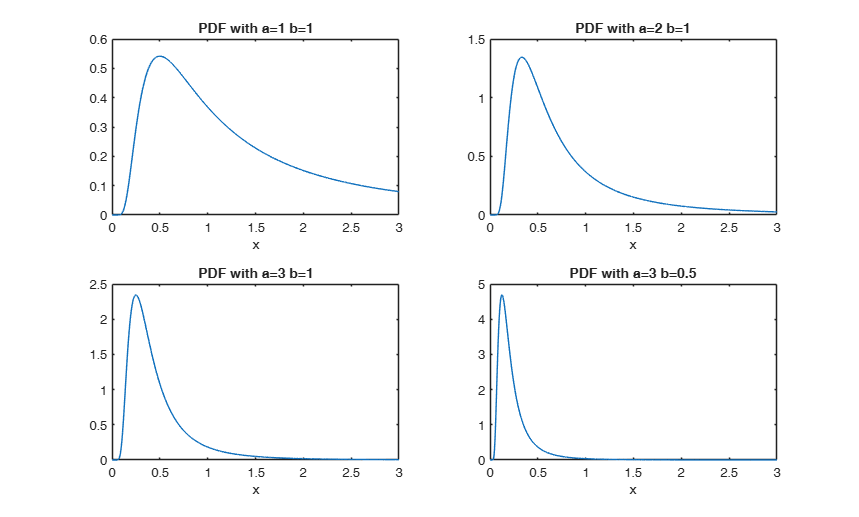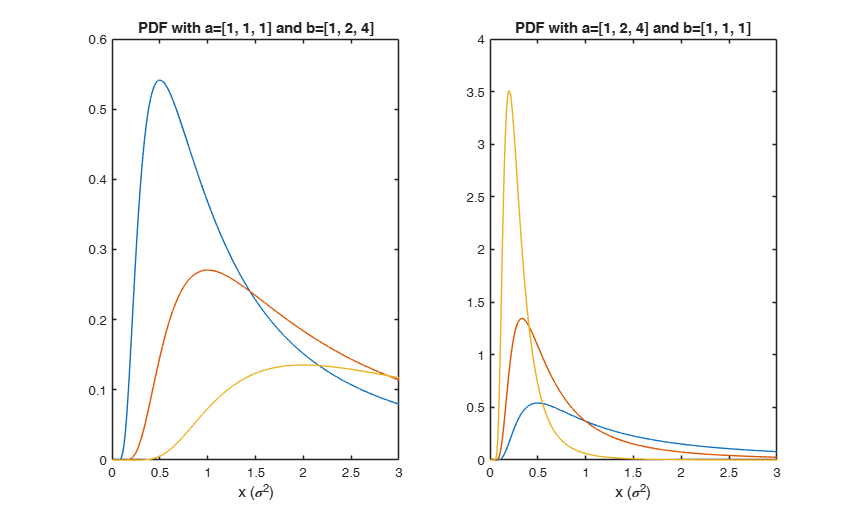# inversegampdf

inversegampdf computes inverse-gamma probability density function.

## Syntax

• y=inversegampdf(x,a,b)example
• y=inversegampdf(x,a,b,nocheck)example

## Description

 y =inversegampdf(x, a, b) Plot the pdf for 4 different combinations of parameter values.

 y =inversegampdf(x, a, b, nocheck) Compare the results using option nocheck=1.

## Examples

expand all

###Plot the pdf for 4 different combinations of parameter values.

x=(0:0.001:3)';
a=[1,2,3,3];
b=[1,1,1,0.5];
for j=1:4
subplot(2,2,j);
plot(x,inversegampdf(x,a(j),b(j)));
xlabel('x');
title(['PDF with a=' num2str(a(j)) ' b=' num2str(b(j))]);
end###Compare the results using option nocheck=1.

x=(0:0.001:3)';
a=[1,2,3,50,100,10000];
b=[1,10,100,0.05,10,800];
Y=zeros(length(x),length(a));
Ychk=Y;
for i=1:length(x)
Y(i,:)   = inversegampdf(x(i),a,b);
Ychk(i,:)= inversegampdf(x(i),a,b,1);
end
disp('Maximum absolute difference is:');
disp(max(max(abs(Y-Ychk))));
Maximum absolute difference is:
2.6049e-09



## Related Examples

expand all

###Interpretation in Bayesian statistics.

Interpretation of a inverse Gamma (conjugate) prior, used for estimating the posterior distribution of the unknown variance $\sigma{^2}$ of a normal $N(0,\sigma{^2})$.

% a set of values for $\sigma^2$
x=(0:0.001:3)';
% Two panels with inverse Gamma distribution for different parameters
% settings.
% Left panel:  fixed shape (1), increasing scale (1,2,4);
% As the scale parameter increases, the mean of the distribution (more
% and more skewed to the right) also increases. This suggests that an
% inverse Gamma prior with a larger scale parameter incorporates a prior
% belief in favour of a larger value for $\sigma^2$.
a = [1, 1, 1];
b = [1, 2, 4];
subplot(1,2,1);
for j=1:3
plot(x,inversegampdf(x,a(j),b(j)));
hold on;
xlabel('x (\sigma^2)');
end
title('PDF with a=[1, 1, 1] and b=[1, 2, 4]');
% Right panel: fixed scale (1), increasing shape (1,2,4);
% As the shape parameter increases, the distribution becomes more and
% more centered around the mean, producing a tighter set of prior beliefs.
b = [1, 1, 1];
a = [1, 2, 4];
subplot(1,2,2);
for j=1:3
plot(x,inversegampdf(x,a(j),b(j)));
hold on;
xlabel('x (\sigma^2)');
end
title('PDF with a=[1, 2, 4] and b=[1, 1, 1]');## Input Arguments

### x — Value at which the pdf must be evaluated. Scalar, vector or matrix 3D array of the same size of x and b.

A scalar input functions as a constant matrix of the same size as the other input.

Data Types: single | double

### a — shape parameter of the inverse-gamma distribution. Scalar, vector or matrix 3D array of the same size of x and b.

A scalar input functions as a constant matrix of the same size as the other input.

Data Types: single | double

### b — scale parameter b of the inverse-gamma distribution. Scalar, vector or matrix 3D array of the same size of x and a.

A scalar input functions as a constant matrix of the same size as the other input.

Data Types: single | double

### nocheck — Check input arguments. Scalar.

If nocheck is equal to 1 no check is performed and input and the density is evaluated directly through the expression y = (b.^a).*(x.^(-a-1)).*exp(-b./x)./gamma(a) else we use MATLAB function gampdf.

Example: 'nocheck',1 

Data Types: double

## Output Arguments

### y —Inverse-gamma pdf value.  Scalar, vector or matrix or 3D array of the same size of input arguments x, a and b

$y=f_{IG}(x | a,b)$ is the value of the pdf of the inverse gamma distribution evaluated at x.

The density of the inverse gamma distribution defined over the support $x>0$ with shape parameter $a$ and scale parameter $b$ is $f_{IG}(x, a, b) \propto x^{-a -1} \exp (-b/x) \frac{b^a}{\Gamma(a)}$

inversegampdf computes the gamma pdf at each of the values in x using the corresponding shape parameters in a and scale parameters in b. Parameters x, a, and b can be vectors, matrices, or multidimensional arrays that all have the same size. A scalar input is expanded to a constant array with the same dimensions as the other inputs. The parameters in a and b must all be positive and the values in x must be in the interval $[0,\infty)$.

The chief use of the inverse gamma distribution is in Bayesian statistics, where the distribution arises as the marginal posterior distribution for the unknown variance of a normal distribution if an uninformative prior is used; and as an analytically tractable conjugate prior if an informative prior is required. See the last example below.

Relation with the Gamma distribution.

If $X \sim Gamma(a,b)$ then $\frac{1}{X} \sim$ inverse-gamma distribution with paramters $a$ and $1/b$.

See the appendix of Zellner (1971) for a detailed description of the inverse Gamma distribution.

Zellner, A. (1971), "An introduction to Bayesian Inference in Econometrics", Wiley.